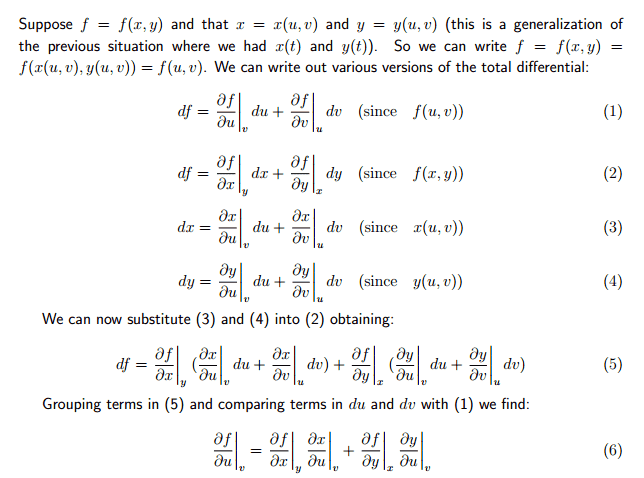# Change of variables/total differential derivation

## Homework Statement

http://gyazo.com/e7dc83f3f93d01391663d02bf92c0b26

## Homework Equations

I am trying to derive equation 6 from 5 using 1, but I haven't got anywhere. This isn't a homework question, just an information sheet. Can someone show me the steps (no jumps please) on obtaining equation 6. After that, for practice I will attempt df/dv

Thanks
:)

SammyS
Staff Emeritus
Science Advisor
Homework Helper
Gold Member

## Homework Statementhttp://gyazo.com/e7dc83f3f93d01391663d02bf92c0b26

## Homework Equations

I am trying to derive equation 6 from 5 using 1, but I haven't got anywhere. This isn't a homework question, just an information sheet. Can someone show me the steps (no jumps please) on obtaining equation 6. After that, for practice I will attempt df/dv

Thanks
:)
It's pretty basic.

Group the terms in (5):
First distribute $\displaystyle \left.\frac{\partial f}{\partial x}\right|_y\text{ and }\left.\frac{\partial f}{\partial y}\right|_x\ .$θ

Then factor out du & dv .

$\displaystyle df=\left( \left.\frac{\partial f}{\partial x}\right|_y \left.\frac{\partial x}{\partial u}\right|_v +\left.\frac{\partial f}{\partial y}\right|_x \left.\frac{\partial y}{\partial u}\right|_v \right)du +\left( \left.\frac{\partial f}{\partial x}\right|_y \left.\frac{\partial x}{\partial v}\right|_u +\left.\frac{\partial f}{\partial y}\right|_x \left.\frac{\partial y}{\partial v}\right|_u \right)dv$

#### Attachments

It's pretty basic.

Group the terms in (5):
First distribute $\displaystyle \left.\frac{\partial f}{\partial x}\right|_y\text{ and }\left.\frac{\partial f}{\partial y}\right|_x\ .$θ

Then factor out du & dv .

$\displaystyle df=\left( \left.\frac{\partial f}{\partial x}\right|_y \left.\frac{\partial x}{\partial u}\right|_v +\left.\frac{\partial f}{\partial y}\right|_x \left.\frac{\partial y}{\partial u}\right|_v \right)du +\left( \left.\frac{\partial f}{\partial x}\right|_y \left.\frac{\partial x}{\partial v}\right|_u +\left.\frac{\partial f}{\partial y}\right|_x \left.\frac{\partial y}{\partial v}\right|_u \right)dv$

I can easily get to the second equation
$\displaystyle df=\left( \left.\frac{\partial f}{\partial x}\right|_y \left.\frac{\partial x}{\partial u}\right|_v +\left.\frac{\partial f}{\partial y}\right|_x \left.\frac{\partial y}{\partial u}\right|_v \right)du +\left( \left.\frac{\partial f}{\partial x}\right|_y \left.\frac{\partial x}{\partial v}\right|_u +\left.\frac{\partial f}{\partial y}\right|_x \left.\frac{\partial y}{\partial v}\right|_u \right)dv$

but how does that become equation 6? We've gone from 4 terms to 6 and remove dv and du

Thanks

SammyS
Staff Emeritus
Science Advisor
Homework Helper
Gold Member
I can easily get to the second equation
$\displaystyle df=\left( \left.\frac{\partial f}{\partial x}\right|_y \left.\frac{\partial x}{\partial u}\right|_v +\left.\frac{\partial f}{\partial y}\right|_x \left.\frac{\partial y}{\partial u}\right|_v \right)du +\left( \left.\frac{\partial f}{\partial x}\right|_y \left.\frac{\partial x}{\partial v}\right|_u +\left.\frac{\partial f}{\partial y}\right|_x \left.\frac{\partial y}{\partial v}\right|_u \right)dv$

but how does that become equation 6? We've gone from 4 terms to 6 and remove dv and du

Thanks
All that equation 6 gives is the coefficient of du.

The coefficient of dv is similar.

Compare equation 1 with $\displaystyle df=\left( \left.\frac{\partial f}{\partial x}\right|_y \left.\frac{\partial x}{\partial u}\right|_v +\left.\frac{\partial f}{\partial y}\right|_x \left.\frac{\partial y}{\partial u}\right|_v \right)du +\left( \left.\frac{\partial f}{\partial x}\right|_y \left.\frac{\partial x}{\partial v}\right|_u +\left.\frac{\partial f}{\partial y}\right|_x \left.\frac{\partial y}{\partial v}\right|_u \right)dv\ .$

Awesome. I see :)